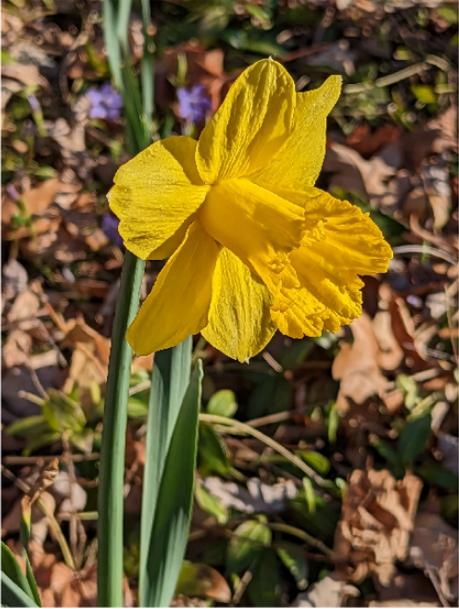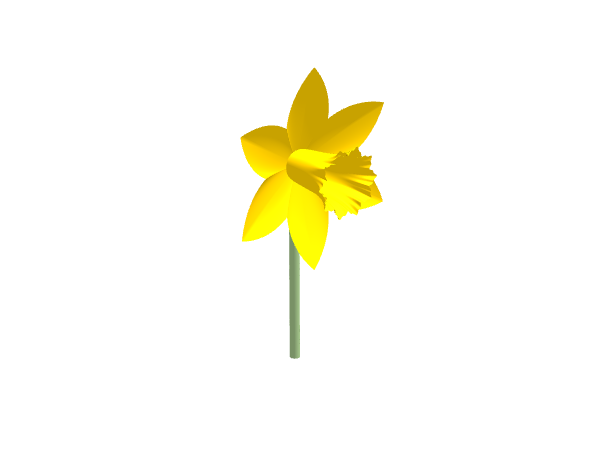# Celebrating springtime: The MATLAB DaffodilAlthough not the earliest to appear here in the UK, Daffodils are the flowers that, for me at least, scream "Spring has sprung." The brief but glorious Daffodil season is coming to an end in my area of the world so I was delighted by the following piece of MATLAB code written by MathWorker Eric Ludlam which allows me to extend the season for a little while longer.
The use of some surprisingly little-known functions that I've written about before: cospi and sinpi also make me happy!
Enjoy!
%% Colors & Sizes
pc = '#faca00'; % petal color
sc = '#97b580'; % stem color
np = 6; % number of petals.
tr = .23; % Radius of trumpet
nruf = 10; % number of trumpet ruffles
sruf = .03; % size of trumpet ruffles
sr = .05; % radius of the stem
%% Unit circle arrays
n = np*50+1; % Theta resolution
theta = linspace(0,2,n);
nr = 20; % Radus resolution
r = linspace(0,1,nr)';
%% Petals
PR = r .* ((1-abs(1-mod(theta*np, 2)))/2+.5);
PX = PR .* cospi(theta);
PY = PR .* sinpi(theta);
PZ = r.^3*.05+hypot(PX,PY)*.2;
% Face our flower sideways by swapping Y&Z
surf(PX,PZ,PY,[],'FaceColor',pc,'EdgeColor','none');
%% Trumpet
flute = flip(1-r*.3);
ruffle = ((cospi(theta*nruf)+2)*sruf).*(linspace(0,1,nr)'.^5);
ruffle2 = ((cospi(theta*nruf*3)+2)*sruf/3).*(linspace(0,1,nr)'.^9);
TR = tr*flute + ruffle + ruffle2;
surface(TR.*cospi(theta),r*tr*3.2,TR.*sinpi(theta),'FaceColor',pc,'EdgeColor','none');
%% Stem
SR = sr .* ones(nr,1);
surface(SR.*cospi(theta),SR.*sinpi(theta)-sr,r*-2.*ones(1,n),'FaceColor',sc,'EdgeColor','none');
%% Configure Axes & Lighting
set(gca,'YDir','reverse','Projection','perspective','Visible','off','DataAspectRatio',[1 1 1])
light('Position',[.5 .5 2],'Color','w');
lighting g
material([.8 .9 .2 2 .4])
view([-40 20]);Perhaps try playing around with the code a little to come up with some variations.
|